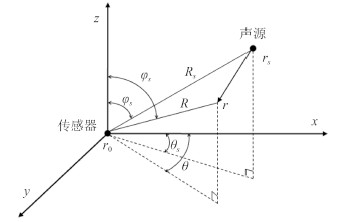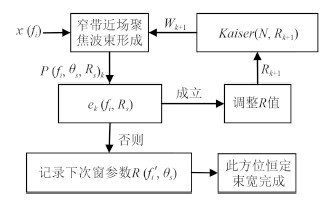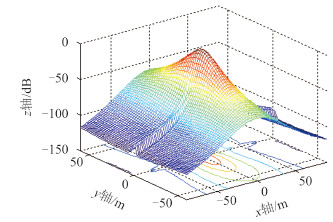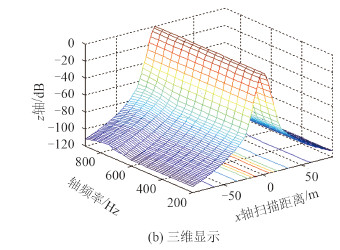﻿ 一种矢量阵近场恒定束宽波束形成方法
 舰船科学技术2016, Vol. 38Issue (3): 111-114PDF

Near-field constant beamwidth beamforming with a vector hydrophone array
WANG Yong-peng, HOU Peng, WANG Jian-wen, XIA Chun-yan
Dalian Scientific Test & Control Technology Institute, Dalian 116013, China
Abstract: An acoustic vector hydrophone, which possesses better directivity, compact geometry and suppression ability of isotropic noise interference, can measure all the three components of the acoustic particle velocity and the pressure at a single point in space, has been proved to be an effective means to improve the measurement ability of ship-radiated noise. Furthermore, with the combination of multiple vector hydrophones, a vector hydrophone array can obtain a higher measurement gain, which helps to evaluate the noise level of weak signatures. In this paper, a near-field signal model of acoustic vector hydrophone is first established, and then two different beamforming methods which utilize the output of a single vector hydrophone or the different types of output of vector hydrophone array are discussed. Aiming at the design problem of constant beamwidth beamformer (CBB), a near-field CBB method with Kaiser weighting is presented. Making use of the characteristics of Kaiser, the designed beam response is gradually converged to the desired beam response and the design precision of CBB is improved with the adoption of exponential verified step. Finally, a computer simulation is conducted to demonstrate the dramatic performance improvement of the proposed algorithm.
Key words: vector hydrophone array    near field    constant beamwidth    beamforming    Kaiser weighting
0 引言

1 矢量阵近场波束形成基础 1.1 近场数学模型的建立图 1 矢量传感器信号模型 Fig. 1 Signal model of single vector hydrophone
 ${a_{near}} = \left[{\begin{array}{*{20}{c}} {\cos {\theta _s}\sin {\phi _s}} \ {\sin {\theta _s}\sin {\phi _s}} \ {\cos {\phi _s}} \ {\frac{{ - {\rho _0}c}}{{\sqrt {1 + {{\left( {\frac{\lambda }{{2\pi {R_s}}}} \right)}^2}} }}{e^{j{\text{arctan}}\frac{\lambda }{{2\pi {R_s}}}}}} \end{array}} \right]$ (1)

Rs/λ→∞，近场阵列流形将收敛为如下形式：

 ${a_{{\text{far}}}} = \left[{\begin{array}{*{20}{c}} {\cos {\theta _s}\sin {\phi _s}} \ {\sin {\theta _s}\sin {\phi _s}} \ {\cos {\phi _s}} \ { - {\rho _0}c} \end{array}} \right]$ (2)
1.2 矢量阵波束形成方法

1)基于单矢量传感器输出信号处理的波束形成

①子阵各个分量相加再加权延时求和：

 $\begin{gathered} B\left( {{f_c},{\theta _s},{\phi _s},{R_s}} \right) = \hfill \ {\left| {\sum\limits_{n = 1}^N {{w_n}} \left( {p + u + v + w} \right)a{{\left( {{f_c},{\theta _s},{\phi _s},{R_s}} \right)}^{\text{T}}}} \right|^2} \hfill \ \end{gathered}$ (3)

②子阵各个分量相乘再加权延时求和：

 $\begin{gathered} B\left( {{f_c},{\theta _s},{\phi _s},{R_s}} \right) = \hfill \ {\left| {\sum\limits_{n = 1}^N {{w_n}} \left( {p{v_x}{v_y}{v_z}} \right)a{{\left( {{f_c},{\theta _s},{\phi _s},{R_s}} \right)}^{\text{T}}}} \right|^2} \hfill \ \end{gathered}$ (4)

2)基于矢量传感器同类输出分量信号处理的波束形成

①相加性子阵波束形成器的波束图为：

 $\begin{array}{*{20}{l}} {B\left( {{f_c},{\theta _s},{\phi _s},{R_s}} \right) = } \ {\left| {\sum\nolimits_{n = 1}^N {\left( {{w_{np}}p + {w_{n{v_x}}}u + {w_{n{v_y}}}v + {w_{n{v_z}}}w} \right)} } \right. \times } \ {{{\left. {\left. {a{{\left( {{f_c},{\theta _s},{\phi _s},{R_s}} \right)}^{\text{T}}}} \right\}} \right|}^2}} \end{array}$ (5)

②相乘性子阵波束形成器的波束图为：

 $\begin{array}{*{20}{l}} {B\left( {{f_c},{\theta _s},{\phi _s},{R_s}} \right) = } \ {\quad \left| {\left\{ {\sum\nolimits_{{\text{n = 1}}}^{\text{N}} {{w_{np}}} pa{{\left( {{f_c},{\theta _s},{\phi _s},{R_s}} \right)}^{\text{T}}}} \right\} \times } \right.} \ {\quad {{\left. {\left\{ {\sum\nolimits_{{\text{n = 1}}}^{\text{N}} {{w_{n{v_y}}}{v_y}a{{\left( {{f_c},{\theta _s},{\phi _s},{R_s}} \right)}^{\text{T}}}} } \right\}} \right|}^2}} \end{array}$ (6)

 $B = {\left| { \sum\nolimits_{n = 1}^N \left( {{p_i}{v_{{x_i}}}} \right) \sum\nolimits_{n = 1}^N {p_i}} \right|^2}$ (7)
 $B = {\left| {\sum\nolimits_{n = 1}^N {\left( {{p_i}\left( {{v_{{x_i}}} + {v_{{y_i}}} + {v_{{z_i}}}} \right)} \right)} \sum\nolimits_{n = 1}^N {{p_i}} } \right|^2}$ (8)
 $B = {\left| {\sum\nolimits_{n = 1}^N \left( {{p_i} + {v_{{y_i}}}} \right)\sum\nolimits_{n = 1}^N {v_{{y_i}}}} \right|^2}$ (9)
2 基于 Kaiser 窗的近场宽带恒定束宽波束形成器设计

 $e\left( {{f_i},{R_s}} \right) = \int_{{\theta _1}}^{{\theta _2}} {\left| {P\left( {{f_i},{\theta _s},{R_s}} \right) - {P_0}\left( {{f_0},{\theta _s},{R_s}} \right)} \right|{\text{d}}{\theta _s}}$ (10)
 $P\left( {{f}_{i}},{{\theta }_{s}},{{R}_{s}} \right)={{W}^{\text{T}}}\left( {{f}_{i}},{{\theta }_{s}},{{R}_{s}} \right)p\left( {{f}_{i}},{{\theta }_{s}},{{R}_{s}} \right)$ (11)

 $MSE = \xi \left( {{f_i}} \right) = E\left\{ {e{{\left( {{f_i}} \right)}^2}} \right\}$ (12)

 $\mathop {\min }\limits_{w\left( {{f_n}} \right) \ne 0} \xi \left( {{f_n}} \right),{f_n} \in \left[{{f_l},{f_h}} \right],n = 1,2,\cdots ,K$ (13)图 2 计算加权量的流程图 Fig. 2 Flow chart for calculating the weighting vector

Kaiser 窗有长度参数 N 和形状参数 β 2 个参数。在利用 Kaiser 窗进行恒定束宽设计时，多数选择均匀变化参数的窗函数，研究发现参数均匀变化的窗函数有很多弊端，当参数较小时，较小的参数变化就可引起窗函数值发生较大的变化。如果在恒定束宽处理中应用参数均匀变化的窗函数，必然会导致在较小窗参数下，处理频率相邻循环的波束主瓣变化大，从而引起恒定束宽处理精度差，甚至会超出参考波束要求的误差范围，导致恒定束宽波束形成失败。因此，本文采用窗函数参数变化的步长随指数变化，如下式：

 $\beta = \sum {{\text{ }}^{(N - k)}}$ (14)

1)根据接收信号的工作频率，将矢量阵的工作频段划分为若干个子带，确保每个子带满足窄带条件；

2)选择合适的矢量阵声压和振速组合形式，在每个子带内分别进行波束形成；

3)确定信号参考频率，为使恒定束宽后的波束不出现栅瓣，选取信号最低子带频率为参考频率，将该频率处的波束图当作参考波束图，利用提出的恒定束宽方法分别对各个子带进行恒定束宽处理，得到各个子带的加权系数，并对各个子带进行加权修正；

4)将修正后的权系数对矢量阵输入信号进行加权处理，就可以得到宽带波束输出，实现宽带信号的不失真接收。

3 设计实例图 3 900 Hz 频率处的三维聚焦波束 Fig. 3 3D focused beampattern of 900Hz frequency图 4 近场恒定束宽波束图 Fig. 4 Near-field beampattern of CBB
4 结语

  TICHAVSKY P, WONG K T, ZOLTOWSKI M D. Near-field/far-field azimuth and elevation angle estimation using a single vector hydrophone[J]. IEEE Transactions on Signal Processing, 2001, 49(11):2498-2510.  WU Y I, WONG K T. Acoustic near-field source-localization by two passive anchor-nodes[J]. IEEE Transactions on Aerospace and Electronic Systems, 2012, 48(1):159-169.  HAWKES M, NEHORAI A. Acoustic vector-sensor beamforming and Capon direction estimation[J]. IEEE Transactions on Signal Processing, 1998, 46(9):2291-2304.  HAWKES M, NEHORAI A. Wideband source localization using a distributed acoustic vector-sensor array[J]. IEEE Transactions on Signal Processing, 2003, 51(6):1479-1491.  JAUTAIKIS E P. Linear and adaptive plane wave beamforming with towed array of acoustic vector sensors[D]. NPS Thesis, M.S. Engineering Acoustics, 2007.  王之程, 陈宗岐, 于沨, 等. 舰船噪声测量与分析[M]. 北京:国防工业出版社, 2004.  WU Y I, WONG, K T, LAU S K. The acoustic vector-sensor's near-field array-manifold[J]. IEEE Transactions on Signal Processing, 2010, 58(7):3946-3951.  SMITH K B, VAN LEIJEN A V. Steering vector sensor array elements with linear cardioids and nonlinear hippioids[J]. The Journal of the Acoustical Society of America, 2007, 122(1):370-377.  WU Y I, Wong, K T. A directionally tunable but frequency-invariant beamformer on an acoustic velocity-sensor triad to enhance speech perception[J]. The Journal of the Acoustical Society of America, 2012131(51):3891-3902.  王之海, 王大成, 曾武. 利用Chebyshev窗函数获得恒定束宽加权矩阵的数值算法[J]. 海洋技术, 2009, 28(3):50-53.